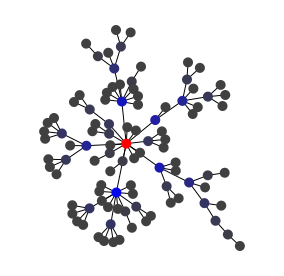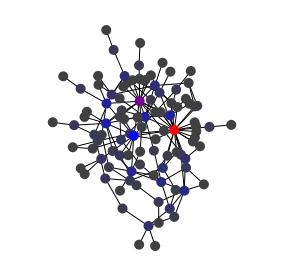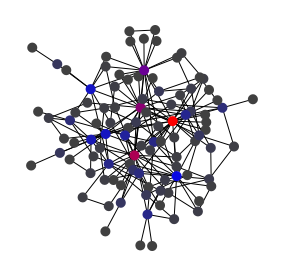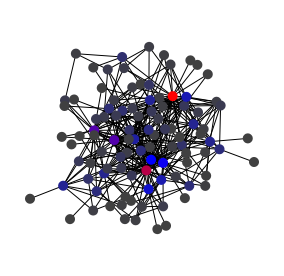# Barabàsi-Albert preferential attachement graph generator

Scale-free graph generator using the preferential attachment rule as defined in the Barabási-Albert model.

This is a very simple graph generator that generates a graph using the preferential attachment rule defined in the Barabási-Albert model: nodes are generated one by one, and each time attached by one or more edges other nodes. The other nodes are chosen using a biased random selection giving more chance to a node if it has a high degree.

## Usage

The more this generator is iterated, the more nodes are generated. It can therefore generate graphs of any size. One node is generated at each call to `nextEvents()`. At each node added at least one new edge is added. The number of edges added at each step is given by the `getMaxLinksPerStep()`. However by default the generator creates a number of edges per new node chosen randomly between 1 and `getMaxLinksPerStep()`. To have exactly this number of edges at each new node, use `setExactlyMaxLinksPerStep(boolean)`.Above are four graphs generated using the Barabàsi-Albert model with four distinct values for the maximum number of links per step, one, two, three and six. Central nodes are highlighted (see the betweenness centrality algorithm).

## Complexity

For each new step, the algorithm act in O(n) with n the number of nodes if 1 max edge per new node is created, else the complexity is O(nm) if m max edge per new node is created.

## Example

Here is an example of use:

## Reference

• Emergence of scaling in random networks,
• Albert-László Barabási & Réka Albert
• Science 286: 509–512.
• October 1999,
• doi:10.1126/science.286.5439.510